Next: Harmonic Oscillators Up: Applications of Statistical Thermodynamics Previous: General Paramagnetism

# Equipartition Theorem

The internal energy of a monatomic ideal gas containing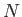particles is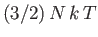. This means that each particle possess, on average,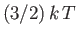units of energy. Monatomic particles have only three translational degrees of freedom, corresponding to their motion in three dimensions. They possess no internal rotational or vibrational degrees of freedom. Thus, the mean energy per degree of freedom in a monatomic ideal gas is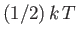. In fact, this is a special case of a rather general result. Let us now try to prove this.

Suppose that the energy of a system is determined by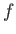generalized coordinates,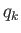, andcorresponding generalized momenta,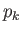, so that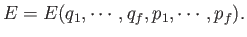(7.124)

Suppose further that:
1. The total energy splits additively into the form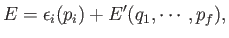(7.125)

where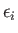involves only one variable,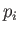, and the remaining part,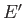, does not depend on.
2. The functionis quadratic in, so that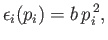(7.126)

where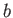is a constant.
The most common situation in which the previous assumptions are valid is whereis a momentum. This is because the kinetic energy is usually a quadratic function of each momentum component, whereas the potential energy does not involve the momenta at all. However, if a coordinate,, were to satisfy assumptions 1 and 2 then the theorem that we are about to establish would hold just as well.

What is the mean value ofin thermal equilibrium if conditions 1 and 2 are satisfied? If the system is in equilibrium at absolute temperature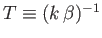then it is distributed according to the canonical probability distribution. In the classical approximation, the mean value ofis expressed in terms of integrals over all phase-space: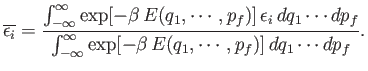(7.127)

Condition 1 gives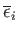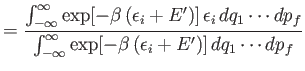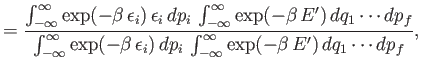(7.128)

where use has been made of the multiplicative property of the exponential function, and where the final integrals in both the numerator and denominator extend over all variables,and, except for. These integrals are equal and, thus, cancel. Hence,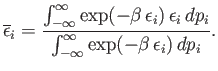(7.129)

This expression can be simplified further because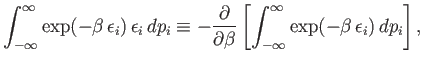(7.130)

so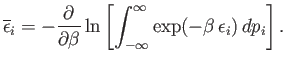(7.131)

According to condition 2,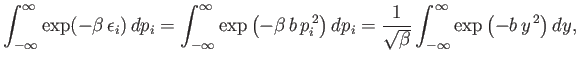(7.132)

where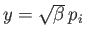. Thus,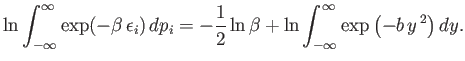(7.133)

Note that the integral on the right-hand side is independent of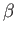. It follows from Equation (7.131) that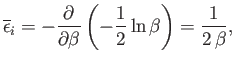(7.134)

giving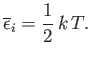(7.135)

This is the famous equipartition theorem of classical physics. It states that the mean value of every independent quadratic term in the energy is equal to. If all terms in the energy are quadratic then the mean energy is spread equally over all degrees of freedom. (Hence, the name equipartition.'')Next: Harmonic Oscillators Up: Applications of Statistical Thermodynamics Previous: General Paramagnetism
Richard Fitzpatrick 2016-01-25